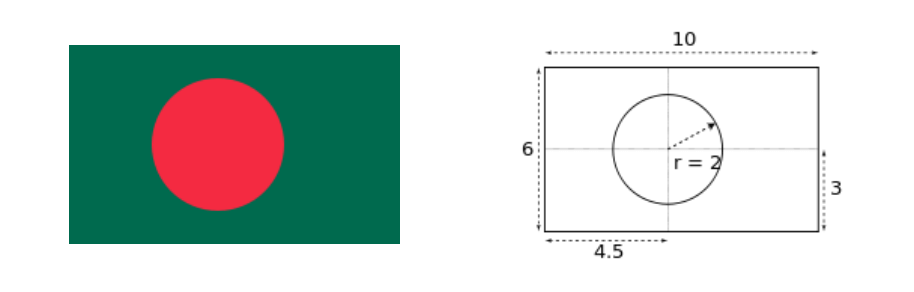Problems
249 - The national flag of Bangladesh (III)

The national flag of Bangladesh (III)

Time Limit: 1 sec

The Problem

The national flag of Bangladesh is bottle green in color and rectangular in size with the length (L) to width(W) ratio of 10:6. It bears a red circle on the background of green. It maintains the length (L) to radius (R) ratio of 5:1. Its centre will be placed on the intersecting point of thee perpendicular drawn from the ninetwentieth part of the length of the flag, and the horizontal line drawn through the middle of its width.

If the lenght is can you find the width (W), radius (R) of the circle and center point (X, Y) of the circle.

The color in the background represents the greenery of Bangladesh while the red circle symbolizes the rising sun and the sacrifice of lives in our freedom fight.

Example:

If the length(L) is 10 then width(W) should be 6, radius(R) should be 2 and center point (X, Y) of the circle is (4.5, 3).The Input

The input file contains only one integer numbers L , which is the length of the flag (L<=50).

The Output

Output will show the lenght(L), the width(W) , radius(R) of red circle and the center point (X, Y) of the circle wich is rounded 1 decimal point of the flag seperated by new line (Seee the sample output).

Sample Input

10

Sample Output

L = 10

W = 6

R = 2

(X, Y) = (4.5, 3.0)

Problem Setter: Shahin ShamS